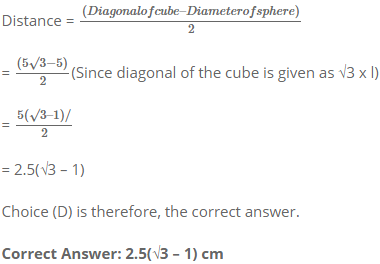Courses

# Test: Mensuration- 2

## 10 Questions MCQ Test Quantitative Aptitude (Quant) | Test: Mensuration- 2

Description
This mock test of Test: Mensuration- 2 for Quant helps you for every Quant entrance exam. This contains 10 Multiple Choice Questions for Quant Test: Mensuration- 2 (mcq) to study with solutions a complete question bank. The solved questions answers in this Test: Mensuration- 2 quiz give you a good mix of easy questions and tough questions. Quant students definitely take this Test: Mensuration- 2 exercise for a better result in the exam. You can find other Test: Mensuration- 2 extra questions, long questions & short questions for Quant on EduRev as well by searching above.
QUESTION: 1

### A right circular cone has height H and radius R. A small cone is cut off at the top by a plane parallel to the base. At what height above the base the section has been made? Statement (I): H = 20 cm Statement (II): Volume of small cone: volume of large cone : 1:15

Solution:

From statement I, we know that the height of the initial cone is 20cm. However, nothing is said about the small cone. Hence, we cannot answer the question using statement A. So, we can eliminate choices (A) and (D).

We are down to choices (A), (B) or (D).

From Statement II, we know that the ratio of the volume of the small cone to that of the large cone is 1 : 15.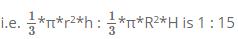(r is the base radius of the smaller cone and h is the height of the smaller cone)
or r2 * h : R2 * H is 1 : 15

From this information, we will not be able to find the answer to h. Hence, we can eliminate choice (A).

Combining the information in the two statements:

When a section is made the two cones are similar triangles. so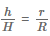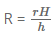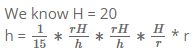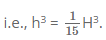Substituting H = 20, we can get the value for h.
Choice (B) is therefore, the correct answer.

Correct Answer: If the question cannot be answered with statement I alone or with statement II alone, but can be answered if both statements are used together.

QUESTION: 2

### A sphere of radius r is cut by a plane at a distance of h from its center, thereby breaking this sphere into two different pieces. The cumulative surface area of these two pieces is 25% more than that of the sphere. Find h.

Solution:

Area = 4πr2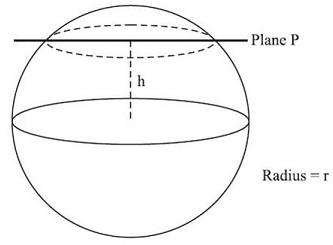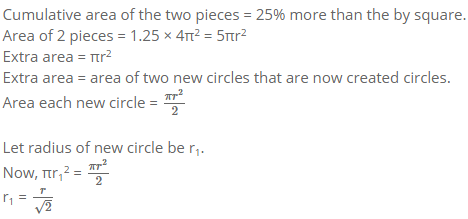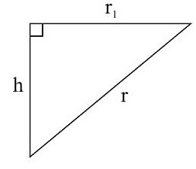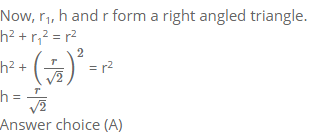QUESTION: 3

### Two mutually perpendicular chords AB and CD meet at a point P inside the circle such that AP = 6 cms, PB = 4 units and DP = 3 units. What is the area of the circle?

Solution: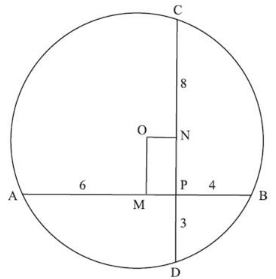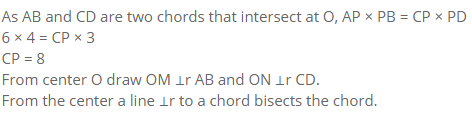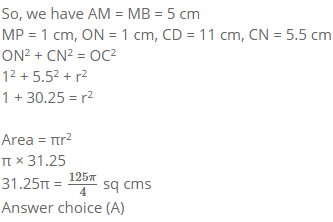QUESTION: 4

Cylindrical cans of cricket balls are to be packed in a box. Each can has a radius of 7 cm and height of 30 cm. Dimension of the box is l = 76 cm, b = 46 cm, h = 45 cm. What is the maximum number of cans that can fit in the box?

Solution:

This question requires a good deal of visualization. Since, both the box and cans are hard solids, simply dividing the volume won’t work because the shape can’t be deformed.

Each cylindrical can has a diameter of 14 cm and while they are kept erect in the box will occupy height of 30 cm

Number of such cans that can be placed in a row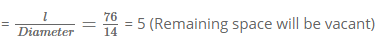Number of such rows that can be placed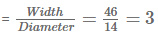Thus 5 x 3 = 15 cans can be placed in an erect position.

However, height of box = 45cm and only 30 cm has been utilized so far

Remaining height = 15 cm > 14 cm (Diameter of the can)

So, some cans can be placed horizontally on the base.

Number of cans in horizontal row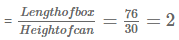Number of such rows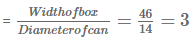∴ 2 x 3 = 6 cans can be placed horizontally

∴ Maximum number of cans = 15+6 = 21

Choice (D) is therefore, the correct answer.

QUESTION: 5

PQRS is a square of sides 2 cm & ST = 2 cm. Also, PT=RT. What is the area of ?PST?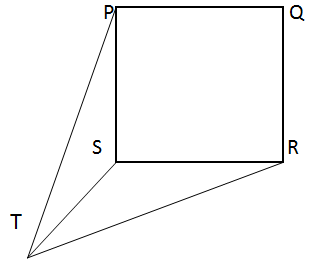Solution:

Although the figure looks like a 3D figure but on reading through the question, it is clear that the diagram is on a single plane.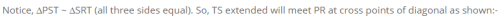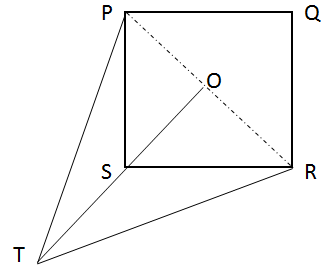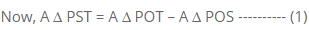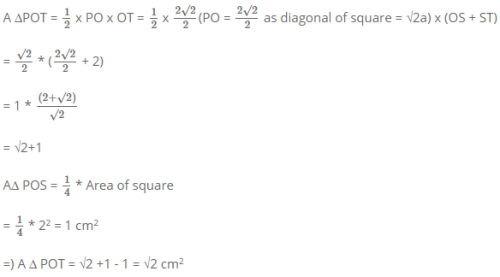QUESTION: 6

A string is wound around two circular disk as shown. If the radius of the two disk are 40 cm and 30 cm respectively. What is the total length of the string?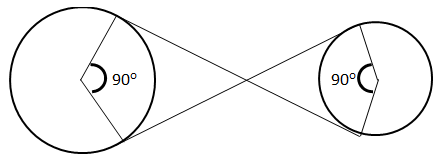Solution: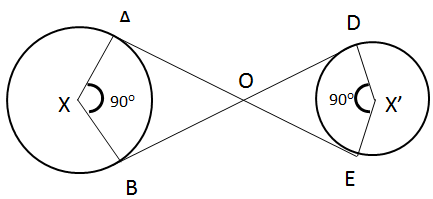As, tangent from an exterior point makes right angle with radius.

=> ADXB and ODXE are squares.

Therefore, AO = BO = 40 cm

OD= DE = 30 cm

Length of string wound around circle x =>    270° / 360°  * 2*π *80

= 3 / 4 * 80 π

= 60 π

Similarly, length of string wound around circle x'

=> 270° / 360° * 2*π*30

=  3 /4 *30π

= 45π /2

Therefore, length of string :-

= ( 40+30+60π+ 45π/2* π)

= 70 + 165π/2

QUESTION: 7

Figure above shows a box which has to be completely wrapped with paper. However, a single Sheet of paper need to be used without any tearing. The dimension of the required paper could be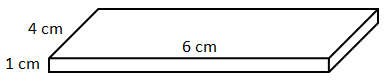Solution:

Total surface area of the box = 2(4x6 + 1x6 + 1x4)

= 2(24 + 6 + 4)

= 68 cm2

As the problem says the paper can’t be torn/cut a portion of paper will need to be fold, so, the area of paper required would be greater than 68 cm2. Only option b) gives the area greater 68 cm2

Choice (B) is therefore, the correct answer.

Correct Answer: 12 cm by 6 cm

QUESTION: 8

An inverted right circular cone has a radius of 9 cm. This cone is partly filled with oil which is dipping from a hole in the tip at a rate of 1cm2/hour. Currently the level of oil 3 cm from top and surface area is 36π cm2. How long will it take the cone to be completely empty?

Solution:

Given,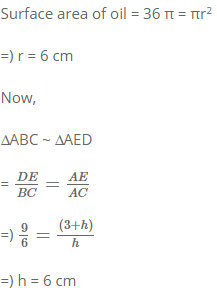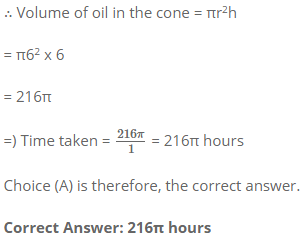QUESTION: 9

A square PQRS has an equilateral triangle PTO inscribed as shown: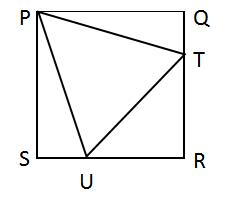What is the ratio of AΔPQT to AΔTRU?

Solution: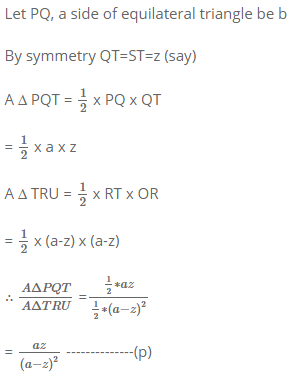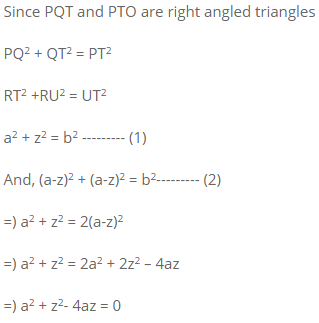=) a2 + z2 – 2az = 2az (Please note how the solution is being managed here. You must always be aware of what you are looking for. Here, as equation -? we are looking for (a-z)2 in terms of az)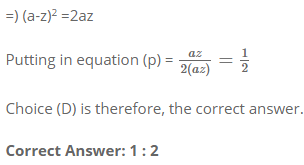QUESTION: 10

A spherical shaped sweet is placed inside a cube of side 5 cm such that the sweet just fits the cube. A fly is sitting on one of the vertices of the cube. What is the shortest distance the fly must travel to reach the sweet?

Solution: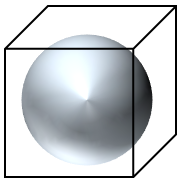The question demands visualization. The shortest distance required to be travelled by the fly would be diagonally and be given by:-